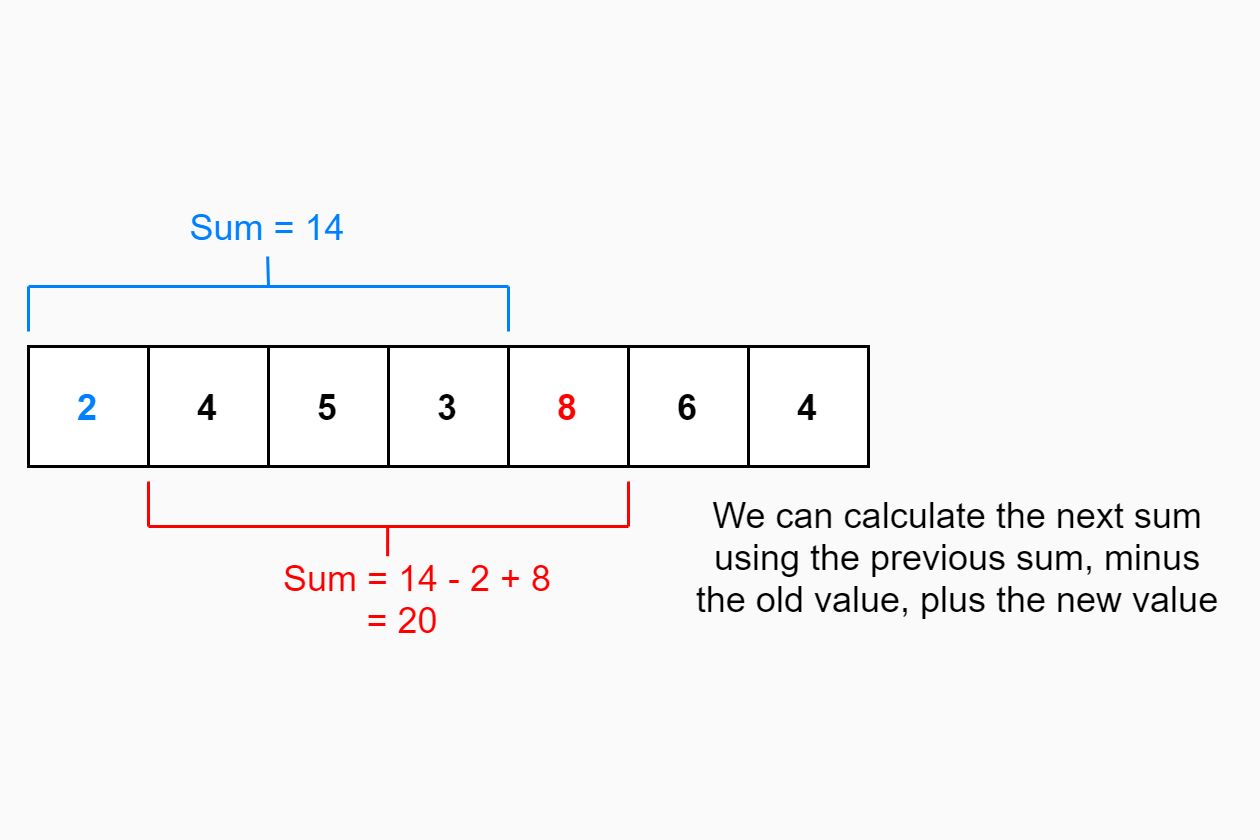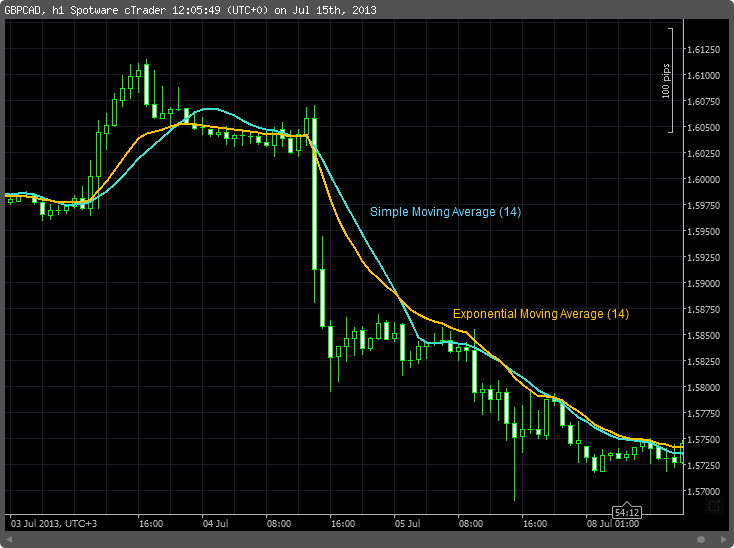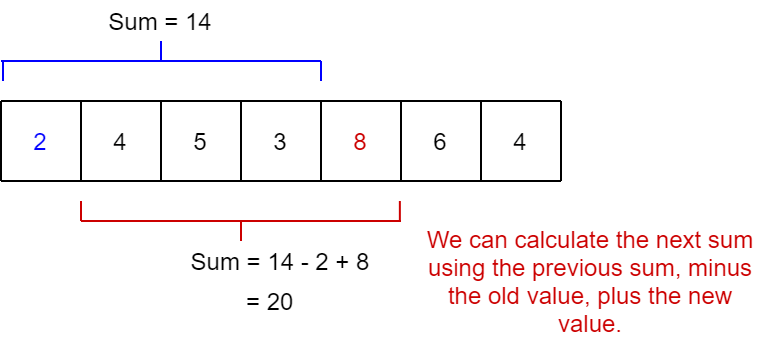# A Simple Moving Average calculator

Share on:

In this post I describe the Simple Moving Average, show a common optimisation for calculating it, and show a C# implementation. Finally I use Spectre.Console to allow calling the calculator, passing in the values and the window size `k`. In subsequent posts I'll expand on this implementation to add extra features and make it thread-safe.

## Background: What is a simple moving average?

A feature I was working on at work the other day required us to keep track of a Simple Moving Average (SMA). The SMA is the mean of the last `k` values, where `k` is specified ahead of time. I find it easiest to work with examples, so in the following table I list the values that we want to average, and the SMA with `k=3`, showing how the SMA was calculated:

ValueSimple Moving AverageCalculation
20.67`(0 + 0 + 2) / 3`
42`(0 + 2 + 4) / 3`
53.67`(2 + 4 + 5) / 3`
34`(4 + 5 + 3) / 3`
85.33`(5 + 3 + 8) / 3`
65.67`(3 + 8 + 6) / 3`
46`(8 + 6 + 4) / 3`

The simple moving average is a way of applying some simple smoothing to a noisy dataset. For example, the following image (taken from Wikipedia) shows a noisy financial data set, with the simple moving average overlaid on top (along with a related average, the exponential moving average).As you can see, the SMA smooths out the noisy source data, though it is not perfect at tracking the real value. We wanted to use the SMA to smooth out some similarly noisy data.

I was following an RFC specification, so the choice of SMA over other moving averages had already been made. For a description of some of the drawbacks of the SMA and alternatives, see Wikipedia.

The naïve implementation of the SMA requires `k-1` additions and a division every time you want to update the value, but we can actually do better than that.

Instead of adding up all the values to get the sum of the last `k` inputs, we can take the previous sum, subtract the oldest value, and add the new value. That gives us the new sum, which we can divide by `3` to get the SMA. That doesn't make much difference when you have a small value of `k`, but for larger values of `k` you can significantly reduce the number of operations you need to do.In both the naïve and alternative approach you still need to keep track of the last `k` inputs

That covers the background of the SMA, so how can we implement this in C#?

## Creating a Simple Moving Average in C#

An initial implementation for the simple moving average is shown below. In this example you create an instance of the `SimpleMovingAverage` class, specifying the value of `k`, i.e. the number of time points over which you want to calculate the SMA. You can then call `Update()`, passing in the new input.

``````public class SimpleMovingAverage
{

private int _index = 0;
private int _sum = 0;

public SimpleMovingAverage(int k)
{
if (k <= 0) throw new ArgumentOutOfRangeException(nameof(k), "Must be greater than 0");

_k = k;
_values = new int[k];
}

public double Update(int nextInput)
{
// calculate the new sum
_sum = _sum - _values[_index] + nextInput;

// overwrite the old value with the new one
_values[_index] = nextInput;

// increment the index (wrapping back to 0)
_index = (_index + 1) % _k;

// calculate the average
return ((double) _sum) / _k;
}
}
``````

In this implementation we use a ring buffer to hold the previous `k` values, and a moving `_index` to point at the next value to remove. Once the buffer fills up (after `k` values), the pointer wraps back round to the start.

We can use this class to perform the SMA calculation on the values I showed at the start of this post with a simple console application:

``````var calculator = new SimpleMovingAverage(k: 3);

var input = new[] {2, 4, 5, 3, 8, 6, 4};

foreach (var value in input)
{
var sma = calculator.Update(value);
Console.WriteLine(\$"The next value is {sma}");
}
``````

Alternatively, we can create a prettier version using Spectre.Console which is functionally identical:

``````using Spectre.Console;

class Program
{
static void Main(string[] args)
{
var calculator = new SimpleMovingAverage(k: 3);

var table = new Table()

var input = new[] {2, 4, 5, 3, 8, 6, 4};
foreach (var value in input)
{
var sma = calculator.Update(value);
}

AnsiConsole.Render(table);
}
}
``````

But which looks nicer when we run it:

``````> dotnet run
┌───────┬───────────────────────┐
│ Value │ Simple Moving Average │
├───────┼───────────────────────┤
│ 2     │ 0.67                  │
│ 4     │ 2.00                  │
│ 5     │ 3.67                  │
│ 3     │ 4.00                  │
│ 8     │ 5.33                  │
│ 6     │ 5.67                  │
│ 4     │ 6.00                  │
└───────┴───────────────────────┘
``````

Lets go one better and allow you to provide the input values on the command line using Spectre.Console.Cli. For this we define a settings class for holding the input and setting the value of `k`:

``````public class SimpleMovingAverageSettings : CommandSettings
{
[CommandArgument(0, "[INPUT]")]
public int[] InputValues { get; set; }

[CommandOption("-k <WINDOW_SIZE>")]
public int K { get; set; } = 3;
}
``````

Next we extract our implementation into a `Command<>` class, and use the injected `SimpleMovingAverageSettings`:

``````public class SimpleMovingAverageCommand : Command<SimpleMovingAverageSettings>
{
public override int Execute(CommandContext context, SimpleMovingAverageSettings settings)
{
var calculator = new SimpleMovingAverage(settings.K);

var table = new Table()

foreach (var value in settings.InputValues)
{
var sma = calculator.Update(value);
}

AnsiConsole.Render(table);

return 0;
}
}
``````

Finally, we update our `Program.Main` to register the `Command<>` and invoke it when requested:

``````class Program
{
static int Main(string[] args)
{
var app = new CommandApp();

app.Configure(config =>
{
});

return app.Run(args);
}
}
``````

We can now call the `sma` command, pass in the values, and easily control the value of `k`:

``````> dotnet run sma 2, 4, 5, 3, 8, 6, 4 -k 3
┌───────┬───────────────────────┐
│ Value │ Simple Moving Average │
├───────┼───────────────────────┤
│ 2     │ 0.67                  │
│ 4     │ 2.00                  │
│ 5     │ 3.67                  │
│ 3     │ 4.00                  │
│ 8     │ 5.33                  │
│ 6     │ 5.67                  │
│ 4     │ 6.00                  │
└───────┴───────────────────────┘
``````

## Limitations

The implementation shown in this post performs well enough, but there's a couple of limitations:

• The `SimpleMovingAverage` class is not thread safe.
• You can only fetch the current SMA value when updating it.
• There's no handling of overflow issues in the summation.

For my use case I needed all of these characteristics, so in the next post I look at a slightly more complex implementation.

## Summary

In this post I described the Simple Moving Average calculation, and showed an example implementation in C#. I showed how you could use this with Spectre.Console to create a simple SMA calculator application. The SMA implementation in this post is very simple, is not thread safe, and does not allow retrieving the current average without updating it. In the next post I address those concernsWant an email when